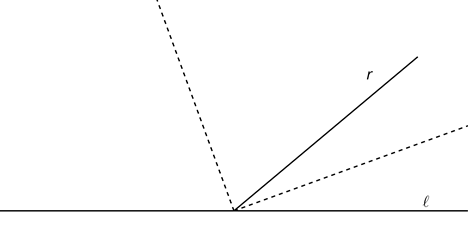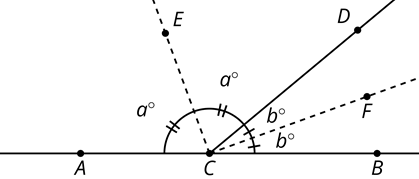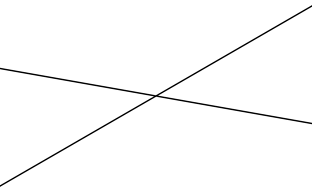# G.1.19.2 That Can’t Be Right, Can It?

Here is a figure where ray r meets line l. The dashed rays are angle bisectors.Diego made the conjecture: “The angle formed between the angle bisectors is always a right angle, no matter what the angle between r and l is.” It is difficult to tell specifically which angles Diego is talking about in his conjecture. Here is a labeled diagram and a rephrasing of Diego's conjecture with more specific details:

• We're given that ray CE bisects angle ACD into two congruent angles that each measure a degrees.
• We're given that ray CF bisects angle DCB into two congruent angles that each measure b degrees.
• Diego concludes that angle ECF is a right angle, in other words a + b = 90 degrees.Use the labeled diagram to explain why Diego's conjecture is true.

Now it's your turn to practice creating a detailed, labeled diagram and rephrasing a conjecture. Here are 2 intersecting lines that create 2 pairs of vertical angles:This is a picture of 2 lines that cross, but we can't discuss the lines becaue there are no labels to refer to. Use the tools below to create a labeled diagram similar to the one above.

Priya writes the conjecture "Vertical angles are congruent". Rephrase Priya's conjecture more precisely using the labels from your diagram above.

Use 180 degree rotations and your labeled diagram and detailed description to explain why Priya's conjecture is true.